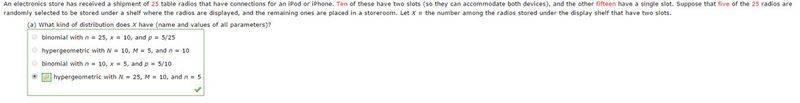# Simple Statistics Question

• Callix
ANS: h(x; 7, 4, 13)Here, M = 4 = number of observed candidates whereas 7 = n = subset within N (13). This is constructed exactly as the term project example I gave first. But both are different to the second example I gave.Rather than start another problem, I suggest you write out what you think the formulas for the answers are for the first two using Combinations notation as in the wiki article. Show what values you have chosen in each case for N, K (M) and n, and what answer you got when you did the calc. I suspect theref

#### Callix

The assignment was already turned in a while ago, but I am currently reviewing all the past homework and trying to resolve the problems I couldn't understand. The website software gives the correct multiple choice or numerical answer, but not the steps. They gave me a weird answer and I didn't think it matched what it should be.

1. Homework Statement

An instructor who taught two sections of statistics last term, the first with 20 students and the second with 35, decided to assign a term project. After all projects had been turned in, the instructor randomly ordered them before grading. Consider the first 15 graded projects.

What is the probability that exactly 10 of these are from the second section?

ANS: 0.2392 where N=55, M=15, n=35

n/a

## The Attempt at a Solution

I disagree with this.. I thought that the number of observations is denoted by n, which should equal 15 because we are observing 15 of the projects. M would be the subset, which, since it's asking with regards to the second section, should be 35. So why is M and n flipped in this scenario??

Later in my homework, one of the solutions to the questions even uses a hypergeometric distribution that specifically shows that n=# of observations and m=amount in a subset of N...
Here is a screenshot of said question:Which question has the wrong "correct" answer then?
Maybe I'm just misinterpreting the questions?

Any help is greatly appreciated! :)

Have a look at the wiki page on hypergeometrics.
It uses N for population size, K for number of successes in population (M in your course's notation), n for sample size and k for number of successes in sample. k is the variable that we say has a hypergeo distribution.

In your first example N=55, K (M) = 35, n=15, k=10. A paper form the 'second section' is a 'success'.
In your 2nd example N=25, K (M) = 10, n=5. A radio with two slots is a 'success'.

Have a look at the wiki page on hypergeometrics.
It uses N for population size, K for number of successes in population (M in your course's notation), n for sample size and k for number of successes in sample. k is the variable that we say has a hypergeo distribution.

In your first example N=55, K (M) = 35, n=15, k=10. A paper form the 'second section' is a 'success'.
In your 2nd example N=25, K (M) = 10, n=5. A radio with two slots is a 'success'.

I'm still a bit confused, because for the first scenario, it says n=35 but you're saying n=15 which is what I originally thought

Ah yes, the answer has M and n switched. But their answer of 0.2392 is correct so presumably they didn't have them switched in the calc. My guess is that it's a typing error in that last bit (which I didn't look at) rather than in the calculated probability.

Did you get 0.2392?

Ah yes, the answer has M and n switched. But their answer of 0.2392 is correct so presumably they didn't have them switched in the calc. My guess is that it's a typing error in that last bit (which I didn't look at) rather than in the calculated probability.

Did you get 0.2392?

Yeah I got that answer. So to be sure, the second question with the screenshot is the one that has the typo?

No. The first answer has the typo.

No. The first answer has the typo.

Hmm.. okay, I'm still a tad bit confused.. The second problem is the only one that is different from the others.

Here's another problem for instance:
A personnel direction interviewing 13 senior engineers for 4 job openings has scheduled 7 interviews for day one and 6 for day two. Assume that the candidates are interviewed in a random order.
a). what is the probability that x of the top 4 candidates are interviewed on the first day?

ANS: h(x; 7, 4, 13)

Here, M = 4 = number of observed candidates whereas 7 = n = subset within N (13). This is constructed exactly as the term project example I gave first. But both are different to the second example I gave.

Rather than start another problem, I suggest you write out what you think the formulas for the answers are for the first two using Combinations notation as in the wiki article. Show what values you have chosen in each case for N, K (M) and n, and what answer you got when you did the calc. I suspect there is an inconsistency in the way you are working through the two problems. But we can't identify the inconsistency without you showing your working.